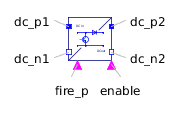Symmetric MultiPhase Cage Winding - MapleSim Help

QuasiStatic Symmetric Multi Phase Cage Winding

Symmetrical rotor cageDescription The QuasiStatic Symmetric MultiPhase Cage Winding component models, using an equivalent symmetrical winding, a cage winding such as that shown below.Connections

 Name Description Modelica ID ${\mathrm{port}}_{p}$ Positive quasi static magnetic port port_p ${\mathrm{port}}_{n}$ Negative quasi static magnetic port port_n $\mathrm{heatPortWinding}$ Heat ports of winding resistor heatPortWindingParameters

 Name Default Units Description Modelica ID $m$ $3$ Number of phases m ${R}_{\mathrm{ref}}$ $\mathrm{\Omega }$ Winding resistance per phase at ${T}_{\mathrm{ref}}$ RRef ${T}_{\mathrm{ref}}$ $293.15$ $K$ Reference temperature of winding TRef $\mathrm{\alpha }$ $0$ $\frac{1}{K}$ Temperature coefficient of winding at 20 degC alpha20 ${T}_{\mathrm{oper}}$ $293.15$ $K$ Operational temperature of winding TOperational ${L}_{\mathrm{\sigma }}$ $H$ Cage stray inductance Lsigma ${n}_{\mathrm{eff}}$ $1$ Effective number of turns effectiveTurns Use Heat Port $\mathrm{false}$ True (checked) means heat port is enabled useHeatPortModelica Standard Library The component described in this topic is from the Modelica Standard Library. To view the original documentation, which includes author and copyright information, click here.